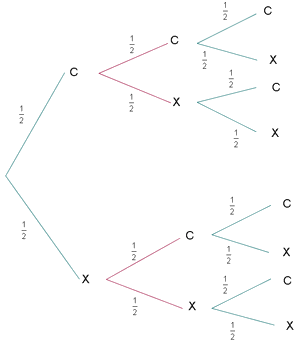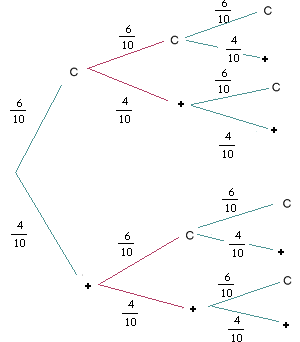# Tree diagrams

The tree diagrams are especially useful to solve problems with compound experiments, that is to say, the ones where we perform more than one random experiment. Some examples of compound experiments are: to throw two coins in the air, and check whether two heads land face up; to say if there are two women out of three siblings; to extract two balls of an urn, and check if there is a red one and a blue one.

Let's consider the following problem:

1. We throw a coin three times into the air. We want to know the probability of the event $$A=$$"to extract two heads".

2. Let's suppose now that the coin has been tampered with, and $$P(C)=\dfrac{6}{10}, P(+)=\dfrac{4}{10}$$ ($$C=$$"heads", $$+=$$"tails"). What is $$P (A)$$ now?

3. To solve the first problem, we can apply the rule of Laplace, since the probability that heads and tails is the result is the same in every toss of the coin, $$1/2$$.

Our sample space is $$\Omega=\{CCC,CC+,C+C,C++,+CC,+C+,++C,+++\}$$.

Favorable cases to $$A$$: $$CCC, CC+, C+C, +CC$$. Therefore, $$P(A)=\dfrac{4}{8}=\dfrac{1}{2}$$.

Let's represent our results in a tree. Starting from the left, in every throw we divide the tree as if it has come out heads $$(C)$$ or tails $$(+)$$, putting on every branch the probability that this happens. In this case, we get quite a simple tree.Every branch of the tree, from the beginning till the end, is a result of the sample space: "first $$C$$ comes out , then $$+$$, and then $$C$$" it corresponds to the elementary event "$$C+C$$".

To compute the probability of every branch, we must multiply the probabilities of all the branches that we have followed till the end of the tree (since this is the probability of the intersection of three independent events). For example, the probability of $$C+C$$ is $$\dfrac{1}{2}\dfrac{1}{2}\dfrac{1}{2}=\dfrac{1}{8}$$.

To solve the problem, we must add the probabilities of all the favorable cases. In our case, every branch, that is to say, every elementary event, has probability $$\dfrac{1}{2}\dfrac{1}{2}\dfrac{1}{2}=\dfrac{1}{8}$$$and there are four favorable branches: $$CCC, CC+, C+C, +CC$$$

Therefore, again we find that $$P(A)=P(CCC)+P(CC+)+P(C+C)+P(+CC)=$$$$$=\dfrac{1}{8}+\dfrac{1}{8}+\dfrac{1}{8}+\dfrac{1}{8}=\dfrac{4}{8}=\dfrac{1}{2}$$$

a probability of $$50\%$$, the same result that we had found earlier. In this case, the tree diagram does not tell us what we already did not know using the Laplace theorem, but let's see what happens in question 2.

1. Now the coin has been tampered with, therefore we cannot directly apply the rule of Laplace. In this case we will see that the use a tree diagram is especially useful.

Let's see our experiment drawn in this case:The cases favorable to $$A$$ are, as before, $$CCC, CC+, C+C, +CC$$.

$$P(CCC)=P(C)\cdot P(C) \cdot P(C) = \dfrac{6}{10}\dfrac{6}{10}\dfrac{6}{10}=\dfrac{216}{1000}$$$$$P(CC+)=P(C)\cdot P(C) \cdot P(+) = \dfrac{6}{10}\dfrac{6}{10}\dfrac{4}{10}=\dfrac{144}{1000}$$$

$$P(C+C)=P(C)\cdot P(+) \cdot P(C) = \dfrac{6}{10}\dfrac{4}{10}\dfrac{6}{10}=\dfrac{144}{1000}$$$$$P(+CC)=P(+)\cdot P(C) \cdot P(C) = \dfrac{4}{10}\dfrac{6}{10}\dfrac{6}{10}=\dfrac{144}{1000}$$$

Finally, $$P(A) = P(CCC) + P(CC+) + P(C+C) + P(+CC)=$$$$$=\dfrac{216}{1000}+\dfrac{144}{1000}+\dfrac{144}{1000}+\dfrac{144}{1000}=\dfrac{648}{1000}=0,648$$$

that is to say, $$A$$ has a probability of $$64'8\%.$$